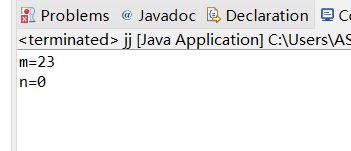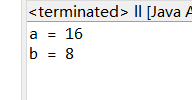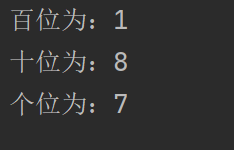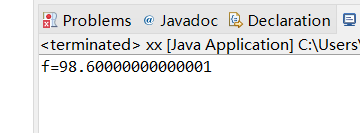# 1903021124—马沙—Java第三周作业—eclipse创建java程序

public class SuanShuDemo {
public static void main(String[] args) {
//声明三个整型变量a、b、c
//分别为a,b,c赋值为4,15,20
//计算(b/a)+c的值 ，并赋值给变量m
//计算(c%b)*a-c的值，并赋值给变量n
//输出m和n的值 } }

package bb;
public class jj {
public static void main(String[] args) {
// TODO Auto-generated method stub
int a;
int b;
int c;
int m,n;
a=4;
b=15;
c=20;
m=(b/a)+c;
n=(c%b)*a-c;
System.out.println("m="+m);
System.out.println("n="+n);
}

}package bb;
public class ll {
public static void main(String[] args) {
// TODO Auto-generated method stub
int a=8 ;
int b=16;
int j=a;
a=b;
b=j;
System.out.println("a = " + a);
System.out.println("b = " + b);
}
}package bb;
public class zz {
public static void main(String[] args) {
// TODO Auto-generated method stub
int j = 187;
int 百位数 = j / 100;
int 十位数 = j % 100/10;
int 个位数 = j % 10;
System.out.println("百位输出为：" + 百位数 );
System.out.println("十位输出为：" + 十位数 );
System.out.println("个位输出为：" + 个位数 );
}

}package bb;

public class xx {
public static void main(String[] args) {
// TODO Auto-generated method stub
double c;
double f;
c=37;
f=c*1.8+32;
c=(f-32)/1.8;
System.out.println("f=" +f );
}
}1、通过这次学习，更加深入的了解了eclipes软件的使用，了解在编写代码时要在英文的状态下。
2、对Java语言的8种基本类型进一步深入了解其使用时的要求。
3、写完每一句都要记得加上该符号来结尾“；”。

posted @ 2022-03-19 22:52  cocopolaris  阅读(49)  评论(0编辑  收藏  举报